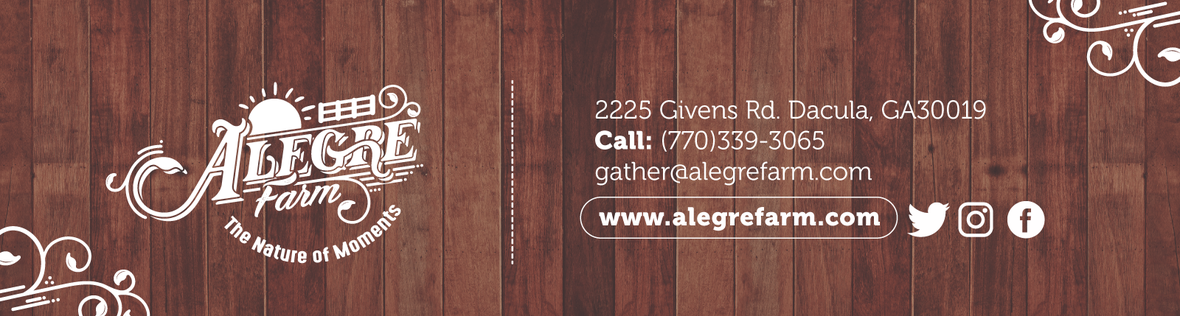Atlanta Real Food is run by the Atlanta area chapter leaders of The Weston A. Price Foundation. Here you will find the latest news from local farmers, get information on how to properly prepare real foods, and stay up to date on local events.

Tuesday, August 14, 2018

Offering Special Days with Discounted Rates from Field Trips to Team Banquets!Hello, Friends!

 table div table+table+table+table div table{width:100%;padding:0}table div table+table+table+table div table img{width:96.23%;padding:0;float:none}table div table+table+table+table div table td{width:100%;padding:0 1.88% 18px}/* styles */Discount Days for Small Groups!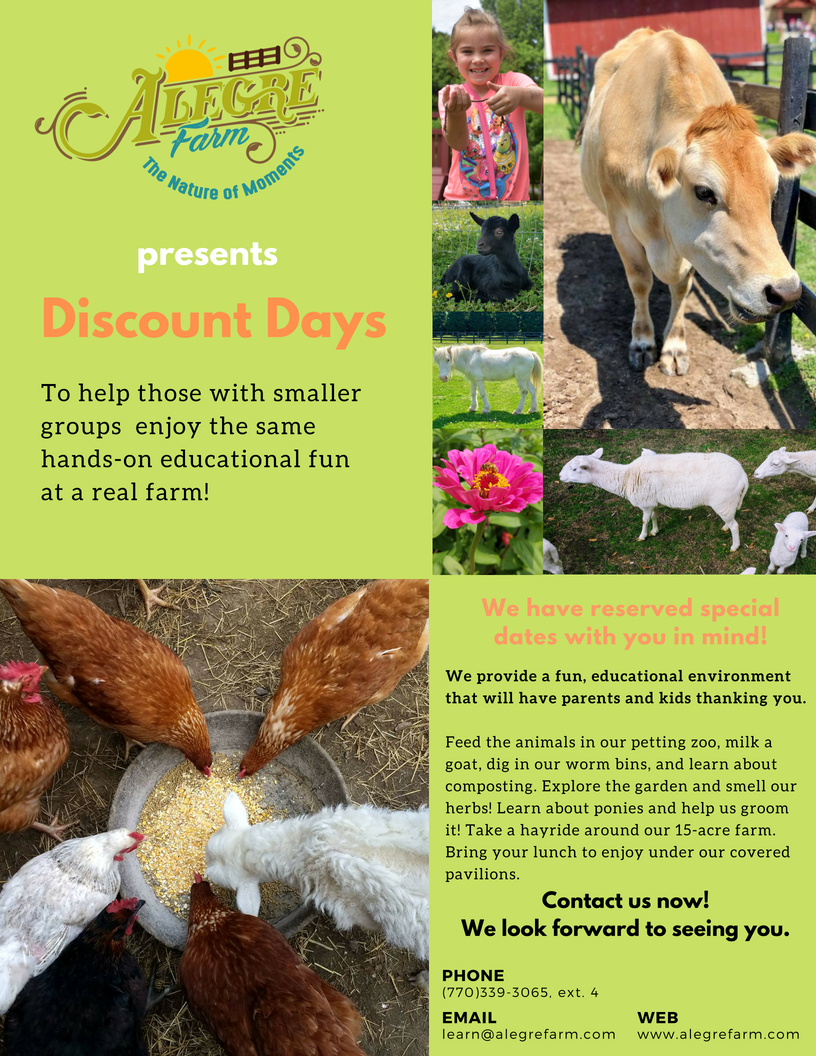Alegre Farm will be having available dates this Fall and Spring season for those who may be homeschooled or have small groups in private schools, daycares, and the like.
This is for those with 18 or less participants to give you the opportunity to enjoy the same fun, educational stations!
Do you know or have a child in a small group that would be interested? Please share this with them.

Phone: (770)339-3065, ext. 4
Email: learn@alegrefarm.com

 table div table+table+table+table+table+table+table div table{width:100%;padding:0}table div table+table+table+table+table+table+table div table img{width:96.23%;padding:0;float:none}table div table+table+table+table+table+table+table div table td{width:100%;padding:0 1.88% 18px}/* styles */Have Your Team Banquet at Alegre Farm!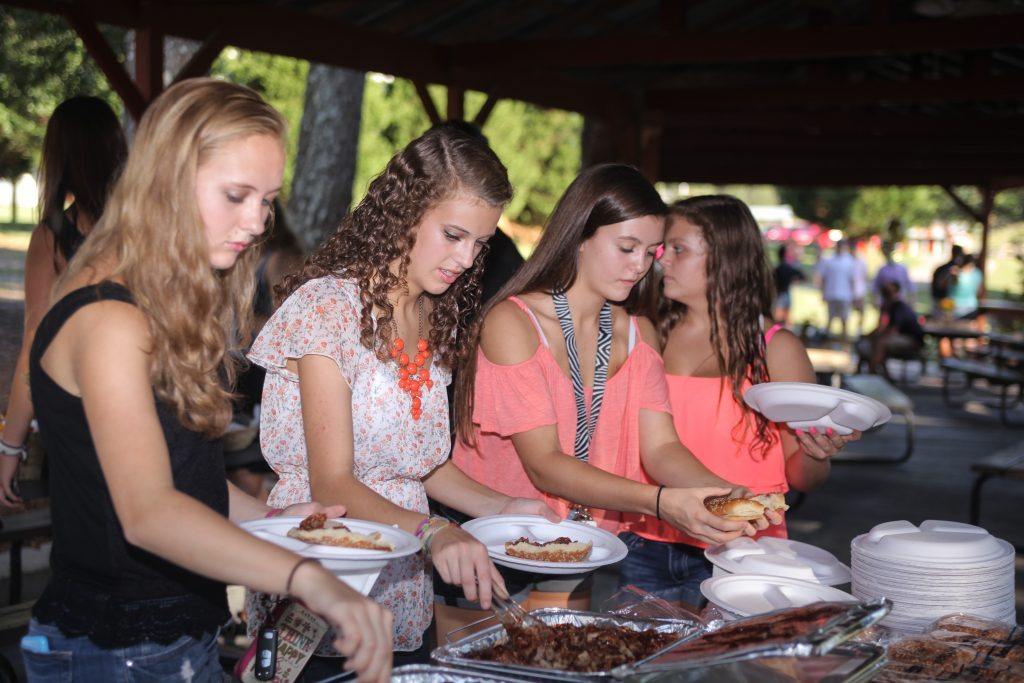It's great to come together as a team and celebrate the hard work that everyone has put in to make the game a successful one!
We have Sundays available from 1-4 PM or 5-8 PM or most weeknights as well to give you special pricing.

You have the option to have your event catered or bring your own food.

* Hayride
* Bonfire with Smores
* Petting Zoo
* Pony Rides
* Popcorn Machine or Sno-Cone Machine
* Bounce House
* Tablecloths
* Projector and Screen
* Amplifier and 2 speakers
* Complete Audio/ Visual Package (includes a projector, screen, amplifier, and 2 speakers)table div table+table+table+table+table+table+table+table+table+table+table+table div table{width:100%;padding:0}table div table+table+table+table+table+table+table+table+table+table+table+table div table img{width:96.23%;padding:0;float:none}table div table+table+table+table+table+table+table+table+table+table+table+table div table td{width:100%;padding:0 1.88% 18px}/* styles */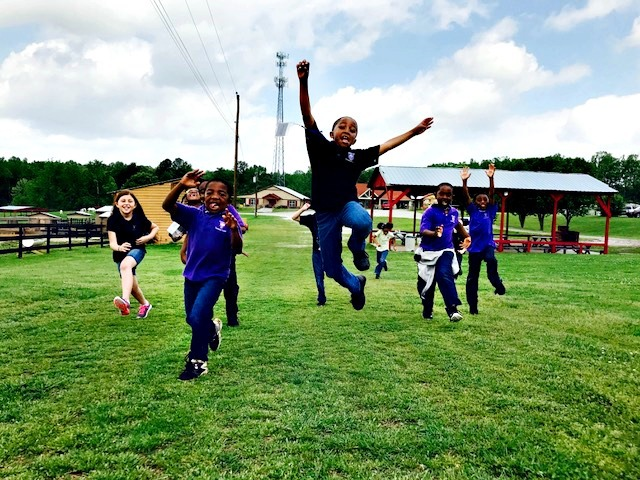We are looking forward to another great school year at Alegre Farm. We offer hands-on field trips where preschool, elementary, middle, and high school students learn about farm life.

We are seeking teachers to guide students through interactive learning stations in our gardens and with our animals. Teaching and farm experience is helpful, but not required. Please contact our Education Director, Angie Mallard, for more information.

Email address is learn@alegrefarm.com, or you can call at (770) 339-3065, extension 4.

Thank you!

 table div table+table+table+table+table+table+table+table+table+table+table+table+table+table+table+table div table{width:100%;padding:0}table div table+table+table+table+table+table+table+table+table+table+table+table+table+table+table+table div table img{width:96.23%;padding:0;float:none}table div table+table+table+table+table+table+table+table+table+table+table+table+table+table+table+table div table td{width:100%;padding:0 1.88% 18px}/* styles */Fall Garden Class Begins Thursday!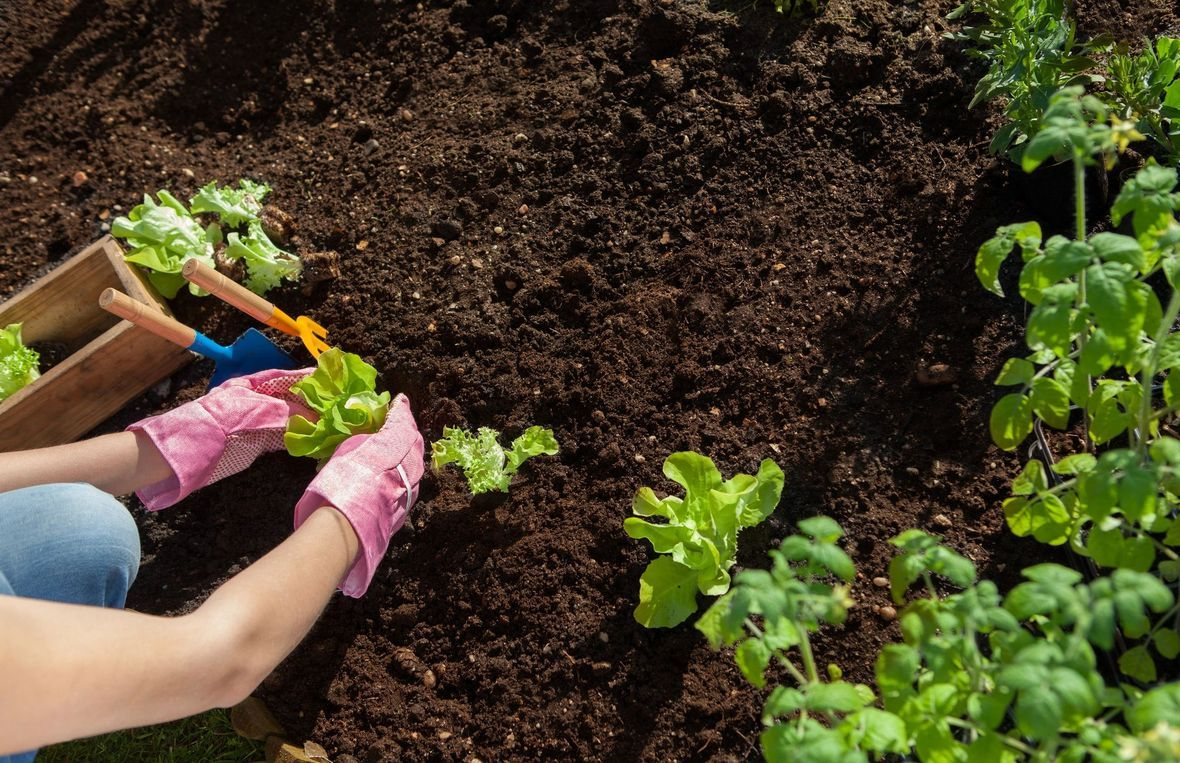Even you can garden and garden right!

3 spots left!

This is your chance to learn how to start your garden or improve and get the most of your garden! If you are a newbie, don't be shy! You will learn so much from Tony, a lead instructor at Gwinnett Tech.

This awesome workshop series is only \$79 and will include 4 classes that are 2 hours each on the following dates from 6pm to 8pm:

August 16
August 23
August 30
September 6

This will be held at Gwinnett Technical College.

 /* styles */ For additional information call or email Tony Gobert at 770-480-9887 or agobert@gwinnetttech.edu
 table div table+table+table+table+table+table+table+table+table+table+table+table+table+table+table+table+table+table+table+table+table div table{width:100%;padding:0}table div table+table+table+table+table+table+table+table+table+table+table+table+table+table+table+table+table+table+table+table+table div table img{width:96.23%;padding:0;float:none}table div table+table+table+table+table+table+table+table+table+table+table+table+table+table+table+table+table+table+table+table+table div table td{width:100%;padding:0 1.88% 18px}/* styles */Garden News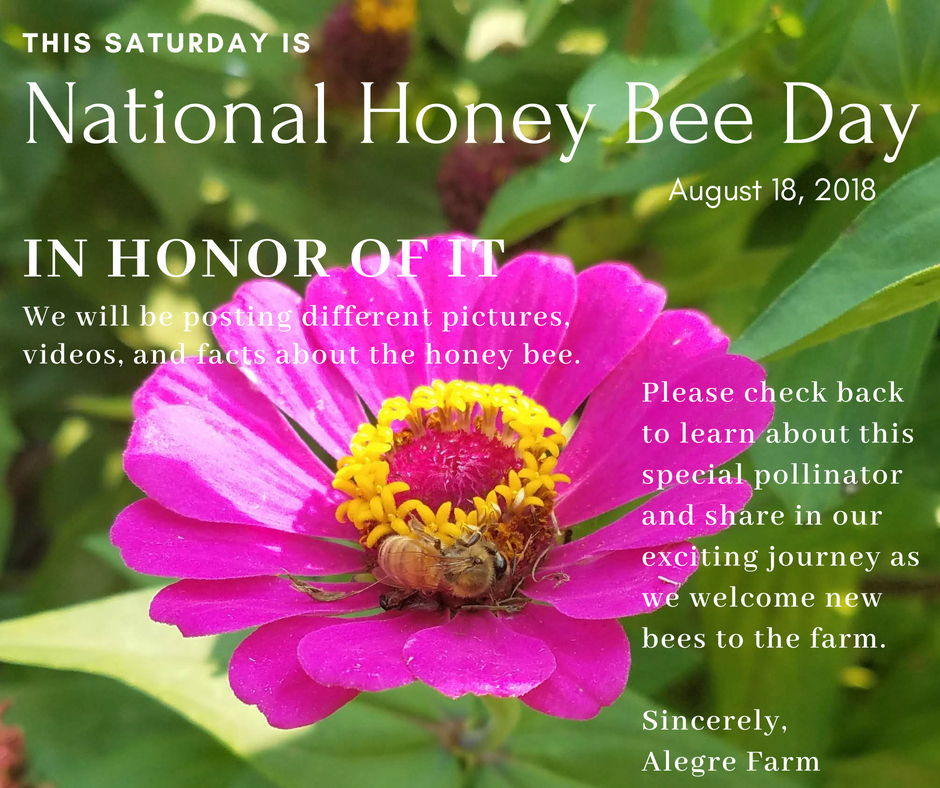We love honey bees in our garden!

This Saturday is National Honey Bee Day! We celebrate this day because the honey bee plays such an important role in our gardens! As it buzzes from flower to flower, it helps pollinate so that we can have delicious fruits and veggies to eat.

 table div table+table+table+table+table+table+table+table+table+table+table+table+table+table+table+table+table+table+table+table+table+table+table+table+table div table{width:100%;padding:0}table div table+table+table+table+table+table+table+table+table+table+table+table+table+table+table+table+table+table+table+table+table+table+table+table+table div table img{width:96.23%;padding:0;float:none}table div table+table+table+table+table+table+table+table+table+table+table+table+table+table+table+table+table+table+table+table+table+table+table+table+table div table td{width:100%;padding:0 1.88% 18px}/* styles */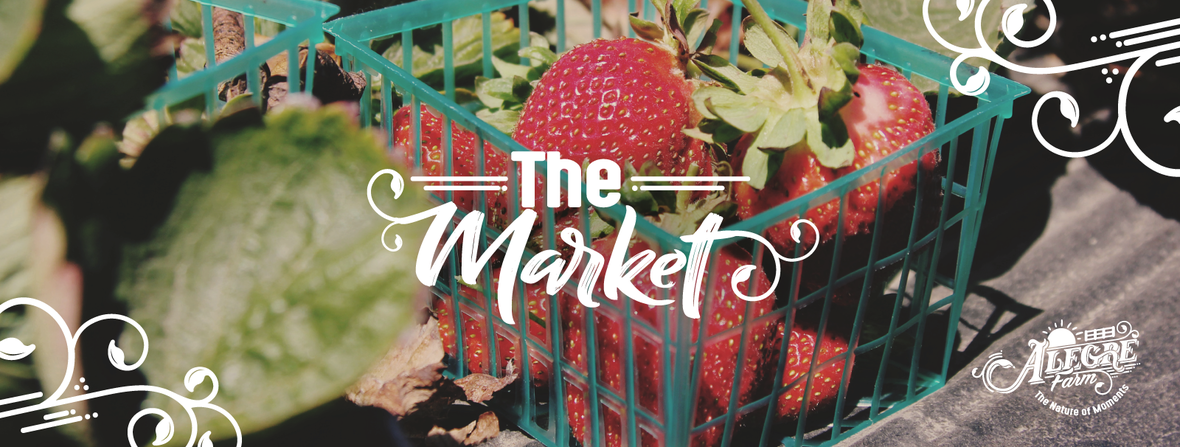Our Online Farmer's Market

We provide the community convenient access to nutrient dense, organically grown foods and healthy products. We are adding new items all the time so please check back weekly.

We will be a delivery site for White Oaks Pasture!

White Oaks Pasture is a multi-generational farm produces Grassfed Beef, Lamb and Goat and Pastured Poultry with traditional sustainable techniques. We are a provider of USDA Inspected pasture raised and hand butchered animals on our Zero Waste, Animal Welfare Approved and Certified Humane Farm.

 table div table+table+table+table+table+table+table+table+table+table+table+table+table+table+table+table+table+table+table+table+table+table+table+table+table+table+table+table+table+table+table div table{width:100%;padding:0}table div table+table+table+table+table+table+table+table+table+table+table+table+table+table+table+table+table+table+table+table+table+table+table+table+table+table+table+table+table+table+table div table img{width:96.23%;padding:0;float:none}table div table+table+table+table+table+table+table+table+table+table+table+table+table+table+table+table+table+table+table+table+table+table+table+table+table+table+table+table+table+table+table div table td{width:100%;padding:0 1.88% 18px}/* styles *//* styles */ Please remember to order by 5pm Wednesdays for the same week pick up.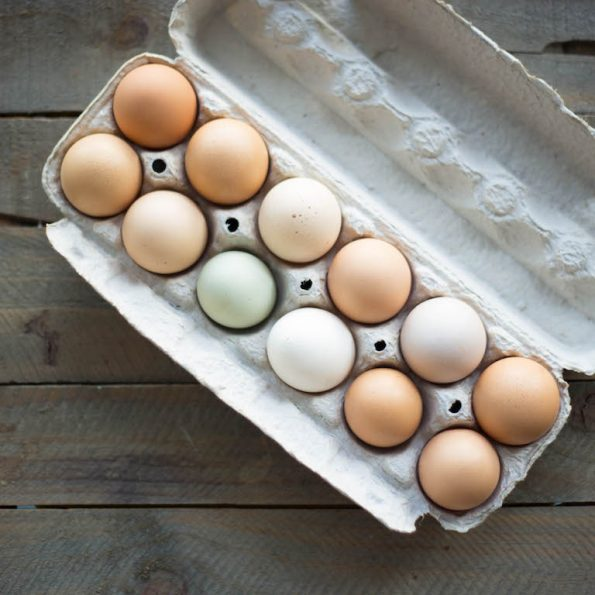Farm Fresh Eggs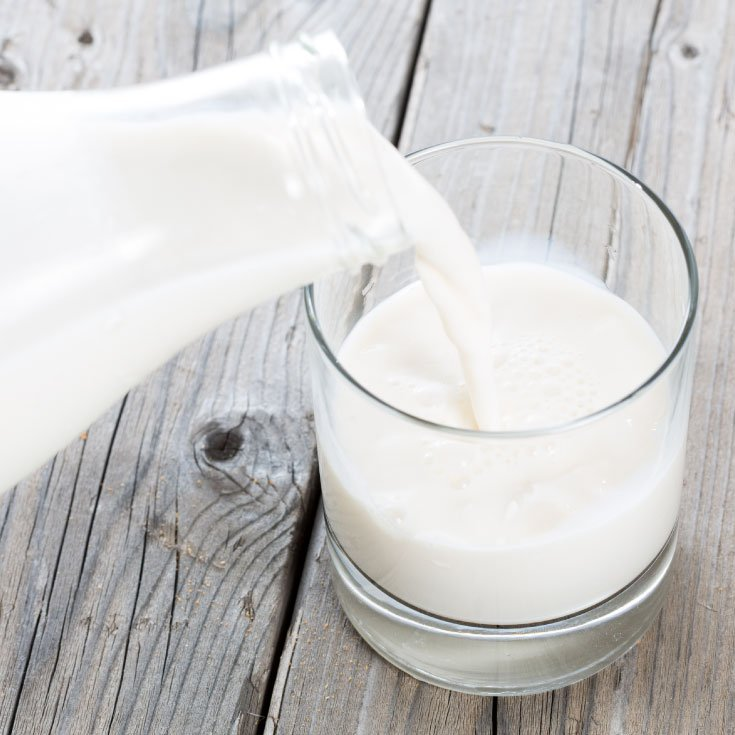Fresh Raw Milk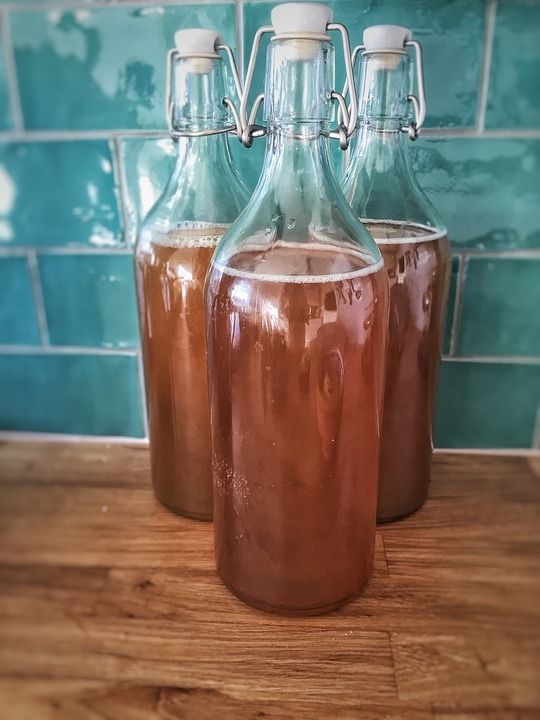Locally Brewed KombuchaAll natural handmade lip balms, soaps, and more!
 table div table+table+table+table+table+table+table+table+table+table+table+table+table+table+table+table+table+table+table+table+table+table+table+table+table+table+table+table+table+table+table+table+table+table+table+table div table{width:100%;padding:0}table div table+table+table+table+table+table+table+table+table+table+table+table+table+table+table+table+table+table+table+table+table+table+table+table+table+table+table+table+table+table+table+table+table+table+table+table div table img{width:96.23%;padding:0;float:none}table div table+table+table+table+table+table+table+table+table+table+table+table+table+table+table+table+table+table+table+table+table+table+table+table+table+table+table+table+table+table+table+table+table+table+table+table div table td{width:100%;padding:0 1.88% 18px}/* styles */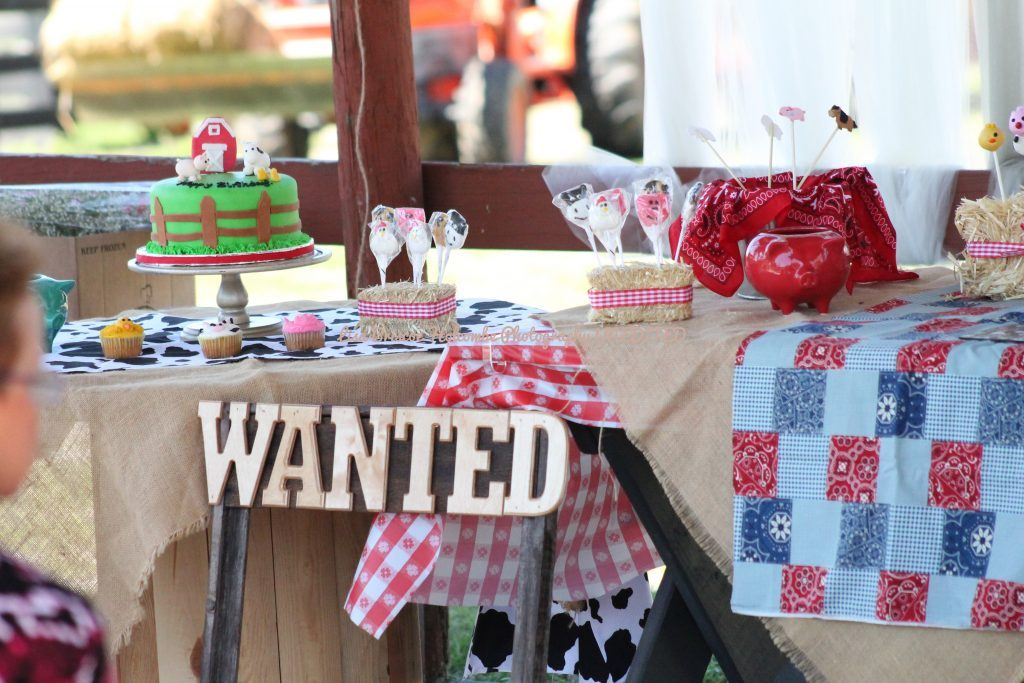Birthday Parties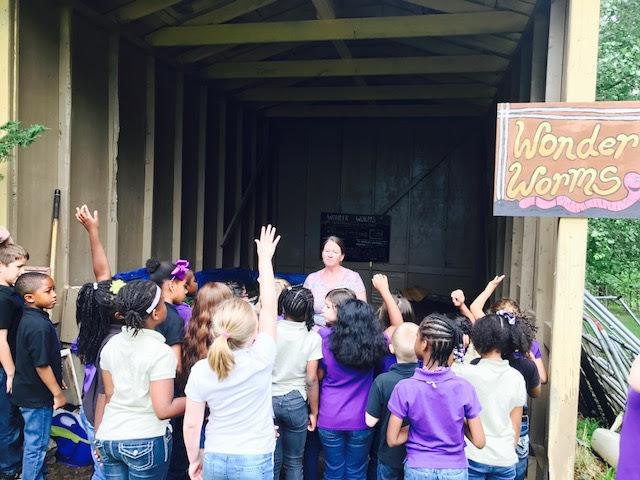Field TripsWeddings
 table div table+table+table+table+table+table+table+table+table+table+table+table+table+table+table+table+table+table+table+table+table+table+table+table+table+table+table+table+table+table+table+table+table+table+table+table+table+table+table+table div table{width:100%;padding:0}table div table+table+table+table+table+table+table+table+table+table+table+table+table+table+table+table+table+table+table+table+table+table+table+table+table+table+table+table+table+table+table+table+table+table+table+table+table+table+table+table div table img{width:96.23%;padding:0;float:none}table div table+table+table+table+table+table+table+table+table+table+table+table+table+table+table+table+table+table+table+table+table+table+table+table+table+table+table+table+table+table+table+table+table+table+table+table+table+table+table+table div table td{width:100%;padding:0 1.88% 18px}/* styles */